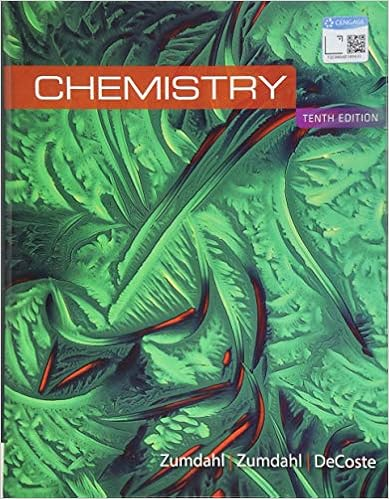• Homework Help
• 11
• 75% (4) 3 out of 4 people found this document helpful

This preview shows page 1 - 3 out of 11 pages.

##### We have textbook solutions for you!
The document you are viewing contains questions related to this textbook.The document you are viewing contains questions related to this textbook.
Chapter 5 / Exercise 93
Chemistry
ZumdahlExpert Verified
AP Chemistry Practice Questions Solids, Liquids and GasesMultiple ChoiceIdentify the choice that best completes the statement or answers the question.____ 1. Which of the following statements is false? a. Condensed states have much higher densities than gases. b. Molecules are very far apart in gases and closer together in liquids and solids. c. Gases completely fill any container they occupy and are easily compressed. d. Vapor refers to a gas formed by evaporation of a liquid or sublimation of a solid. e. Solid water (ice), unlike most substances, is denser than its liquid form (water).
____ 2. Which physical state/ property is incorrectly matched?
____ 3. An open-tube manometer is used to measure the pressure in flask. The atmospheric pressure is 756 torr and the Hg column is 10.5 cm higher on the open end. What is the pressure in the flask?
____ 4. The volume of a sample of a gas is 405 mL at 10.0 atm and 467 K. What volume will it occupy at 4.29 atm and the same temperature?
____ 5. Absolute zero is the temperature at which a. a graph of V versus 1/P intersects the 1/P-axis. b. gaseous helium liquefies. c. the straight line graph of V versus T intersects the T-axis. d. a graph of P versus 1/V intersects the 1/V-axis. e. none of the above
____ 6. A sample of nitrogen occupies 5.50 liters under a pressure of 900. torr at 25.0C. At what temperature will it occupy 10.0 liters at the same pressure?
C
C
C
##### We have textbook solutions for you!
The document you are viewing contains questions related to this textbook.The document you are viewing contains questions related to this textbook.
Chapter 5 / Exercise 93
Chemistry
ZumdahlExpert Verified
____ 7. Snoopy is inflated for the Macy's Thanksgiving parade with 50,000 L of He at 25 C and 1.2 atm. What is the percent decrease in Snoopy's volume if the temperature drops to 4 C before the parade? Assume constant pressure.
____ 8. A gas sample occupies 1.00 L at 120.C and 1.00 atm. What volume will it occupy at STP?
____ 9. A sample of propane, C3H8, occupies 1.73 mL when the pressure is 320 torr at 30C. What is the volume at STP? a. 656 mL b. 499 mL c. 5.09 L d. 3.70 L e. 808 mL
____ 10. What volume will 12.40 grams of CO2occupy at STP, if it behaves ideally?
____ 11. Ten (10.0) moles of a gas are contained in a 10.0 L container at 273 K. Calculate the pressure of the gas.
____ 12. A gaseous compound is 30.4% nitrogen and 69.6% oxygen by mass. A 5.25-gram sample of the gas occupies a volume of 1.00 liter and exerts a pressure of 1.26 atmospheres at - 4.0C. What is its molecular formula?
6
5____
•••